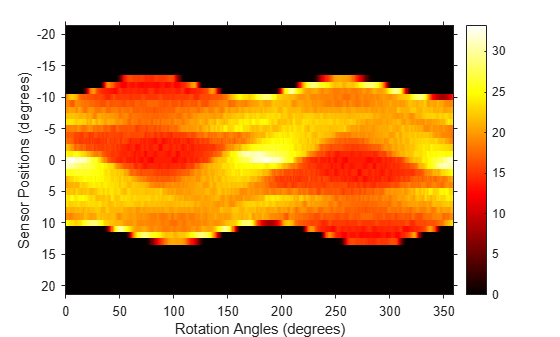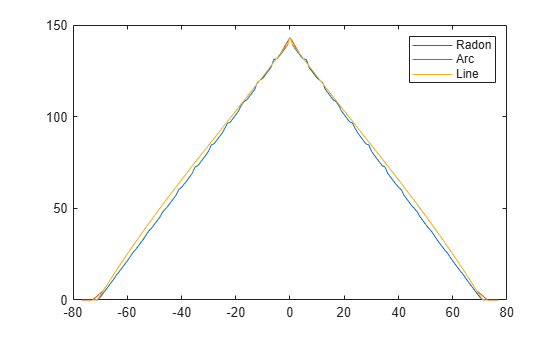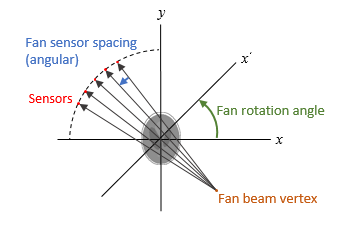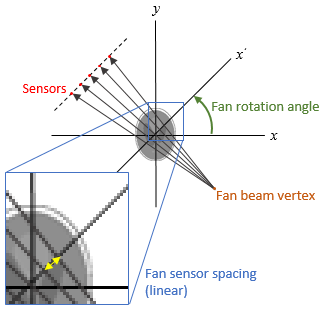# fanbeam

Fan-beam transform

## Syntax

``F = fanbeam(I,D)``
``F = fanbeam(I,D,Name,Value)``
``````[F,fan_sensor_positions,fan_rotation_angles] = fanbeam(___)``````

## Description

example

````F = fanbeam(I,D)` computes the fan-beam projection data (sinogram) `F` from the image `I`. Each column of `F` contains fan-beam projection data at one rotation angle. `D` is the distance from the fan-beam vertex to the center of rotation.```
````F = fanbeam(I,D,Name,Value)` uses name-value pairs to specify the rotation increment and sensor spacing.```

example

``````[F,fan_sensor_positions,fan_rotation_angles] = fanbeam(___)``` returns the location of fan-beam sensors in `fan_sensor_positions` and the rotation angles where the fan-beam projections are calculated in `fan_rotation_angles`.```

## Examples

collapse all

Set the IPT preference to make the axes visible.

`iptsetpref('ImshowAxesVisible','on')`

Create a sample image and display it.

```ph = phantom(128); imshow(ph)```Calculate the fanbeam projections and display them.

```[F,Fpos,Fangles] = fanbeam(ph,250); figure imshow(F,[],'XData',Fangles,'YData',Fpos,... 'InitialMagnification','fit') axis normal xlabel('Rotation Angles (degrees)') ylabel('Sensor Positions (degrees)') colormap(gca,hot), colorbar```Compute fan-beam projections for 'arc' geometry.

```I = ones(100); D = 200; dtheta = 45; [Farc,FposArcDeg,Fangles] = fanbeam(I,D,... 'FanSensorGeometry','arc',... 'FanRotationIncrement',dtheta);```

Convert angular positions to linear distance along x-prime axis.

`FposArc = D*tan(FposArcDeg*pi/180);`

Compute fan-beam projections for 'line' geometry.

```[Fline,FposLine] = fanbeam(I,D,... 'FanSensorGeometry','line',... 'FanRotationIncrement',dtheta);```

`[R,Rpos]=radon(I,Fangles);`

Display the three projections at one particular rotation angle. Note the three are very similar. Differences are due to the geometry of the sampling, and the numerical approximations used in the calculations.

```figure idx = find(Fangles==45); plot(Rpos,R(:,idx),... FposArc,Farc(:,idx),... FposLine,Fline(:,idx)) legend('Radon','Arc','Line')```## Input Arguments

collapse all

Input image, specified as a 2-D numeric or logical matrix.

Distance in pixels from the fan beam vertex to the center of rotation, specified as a positive number. The center of rotation is the center pixel of the image, defined as `floor((size(I)+1)/2)`. `D` must be large enough to ensure that the fan-beam vertex is outside of the image at all rotation angles. See Tips for guidelines on specifying `D`. The figure illustrates `D` in relation to the fan-beam vertex for one fan-beam geometry.### Name-Value Arguments

Specify optional comma-separated pairs of `Name,Value` arguments. `Name` is the argument name and `Value` is the corresponding value. `Name` must appear inside quotes. You can specify several name and value pair arguments in any order as `Name1,Value1,...,NameN,ValueN`.

Example: ```F = fanbeam(I,D,'FanRotationIncrement',5)```

Fan-beam rotation angle increment in degrees, specified as the comma-separated pair consisting of `'FanRotationIncrement'` and a positive scalar.Data Types: `double`

Fan-beam sensor positioning, specified as the comma-separated pair consisting of `'FanSensorGeometry'` and one of the following values.

Value

Meaning

Diagram

`'arc'`

Sensors are spaced at equal angles along a circular arc at distance `D` from the center of rotation.

`FanSensorSpacing` defines the angular spacing in degrees.`'line'`

Sensors are spaced at equal distances along a line that is parallel to the x' axis. The closest sensor is distance `D` from the center of rotation.

`FanSensorSpacing` defines the distance between fan-beams on the x' axis, in pixels.Fan-bean sensor spacing, specified as the comma-separated pair consisting of `'FanSensorSpacing'` and a positive scalar.

• If `FanSensorGeometry` is `'arc'`, then `FanSensorSpacing` defines the angular spacing in degrees.

• If `FanSensorGeometry` is `'line'`, then `FanSensorSpacing` defines the linear distance between fan-beams, in pixels. Linear spacing is measured on the x' axis.

Data Types: `double`

## Output Arguments

collapse all

Fan-beam projection data, returned as a numsensors-by-numangles numeric matrix. numsensors is the number of fan-beam sensors and numangles is the number of fan-beam rotation angles. Each column of `F` contains the fan-beam sensor samples at one rotation angle.

Data Types: `double`

Location of fan-beam sensors, returned as a numsensors-by-1 numeric vector.

• If `FanSensorGeometry` is `'arc'` (the default), then `fan_sensor_positions` contains the fan-beam spread angles.

• If `FanSensorGeometry` is `'line'`, then `fan_sensor_positions` contains the fan-beam sensor positions along the x' axis. See `FanSensorSpacing` for more information.

`fanbeam` determines the number of sensors by calculating how many beams are required to cover the entire image for any rotation angle. Fewer sensors are required to cover the image when the distance `D` between the fan-beam vertex and the center of rotation is large.

Data Types: `double`

Rotation angle of fan-beam sensors, returned as a 1-by-numangles numeric vector. numangles is `360/FanRotationIncrement`.

Data Types: `double`

## Tips

As a guideline, try making `D` a few pixels larger than half the image diagonal dimension, calculated as follows.

`sqrt(size(I,1)^2 + size(I,2)^2)`

The values returned in `F` are a numerical approximation of the fan-beam projections. The algorithm depends on the Radon transform, interpolated to the fan-beam geometry. The results vary depending on the parameters used. You can expect more accurate results when the image is larger, `D` is larger, and for points closer to the middle of the image, away from the edges.

 Kak, A.C., & Slaney, M., Principles of Computerized Tomographic Imaging, IEEE Press, NY, 1988, pp. 92-93.